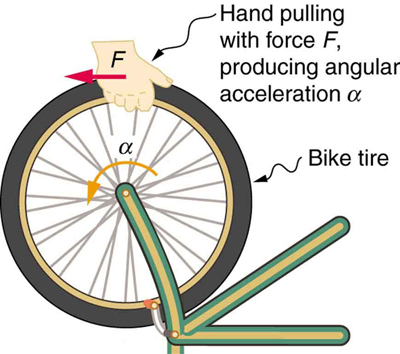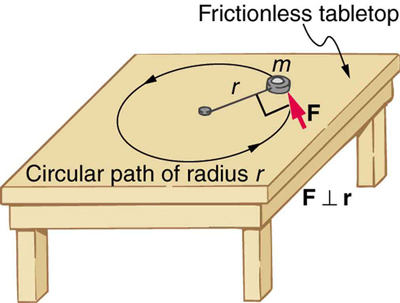# 10.3 Dynamics of rotational motion: rotational inertia

 Page 1 / 8
• Understand the relationship between force, mass and acceleration.
• Study the turning effect of force.
• Study the analogy between force and torque, mass and moment of inertia, and linear acceleration and angular acceleration.

If you have ever spun a bike wheel or pushed a merry-go-round, you know that force is needed to change angular velocity as seen in [link] . In fact, your intuition is reliable in predicting many of the factors that are involved. For example, we know that a door opens slowly if we push too close to its hinges. Furthermore, we know that the more massive the door, the more slowly it opens. The first example implies that the farther the force is applied from the pivot, the greater the angular acceleration; another implication is that angular acceleration is inversely proportional to mass. These relationships should seem very similar to the familiar relationships among force, mass, and acceleration embodied in Newton’s second law of motion. There are, in fact, precise rotational analogs to both force and mass.Force is required to spin the bike wheel. The greater the force, the greater the angular acceleration produced. The more massive the wheel, the smaller the angular acceleration. If you push on a spoke closer to the axle, the angular acceleration will be smaller.

To develop the precise relationship among force, mass, radius, and angular acceleration, consider what happens if we exert a force $F$ on a point mass $m$ that is at a distance $r$ from a pivot point, as shown in [link] . Because the force is perpendicular to $r$ , an acceleration $a=\frac{F}{m}$ is obtained in the direction of $F$ . We can rearrange this equation such that $F=\text{ma}$ and then look for ways to relate this expression to expressions for rotational quantities. We note that $a=\mathrm{r\alpha }$ , and we substitute this expression into $F=\text{ma}$ , yielding

$F=\text{mr}\alpha \text{.}$

Recall that torque    is the turning effectiveness of a force. In this case, because $\mathbf{\text{F}}$ is perpendicular to $r$ , torque is simply $\tau =\mathrm{Fr}$ . So, if we multiply both sides of the equation above by $r$ , we get torque on the left-hand side. That is,

$\text{rF}={\text{mr}}^{2}\alpha$

or

$\tau ={\text{mr}}^{2}\mathrm{\alpha .}$

This last equation is the rotational analog of Newton’s second law ( $F=\text{ma}$ ), where torque is analogous to force, angular acceleration is analogous to translational acceleration, and ${\text{mr}}^{2}$ is analogous to mass (or inertia). The quantity ${\text{mr}}^{2}$ is called the rotational inertia    or moment of inertia    of a point mass $m$ a distance $r$ from the center of rotation.An object is supported by a horizontal frictionless table and is attached to a pivot point by a cord that supplies centripetal force. A force F size 12{F} {} is applied to the object perpendicular to the radius r size 12{r} {} , causing it to accelerate about the pivot point. The force is kept perpendicular to r size 12{r} {} .

## Making connections: rotational motion dynamics

Dynamics for rotational motion is completely analogous to linear or translational dynamics. Dynamics is concerned with force and mass and their effects on motion. For rotational motion, we will find direct analogs to force and mass that behave just as we would expect from our earlier experiences.

derivative of first differential equation
why static friction is greater than Kinetic friction
draw magnetic field pattern for two wire carrying current in the same direction
An American traveler in New Zealand carries a transformer to convert New Zealand’s standard 240 V to 120 V so that she can use some small appliances on her trip.
What is the ratio of turns in the primary and secondary coils of her transformer?
nkombo
How electric lines and equipotential surface are mutually perpendicular?
The potential difference between any two points on the surface is zero that implies È.Ŕ=0, Where R is the distance between two different points &E= Electric field intensity. From which we have cos þ =0, where þ is the angle between the directions of field and distance line, as E andR are zero. Thus
sorry..E and R are non zero...
By how much leeway (both percentage and mass) would you have in the selection of the mass of the object in the previous problem if you did not wish the new period to be greater than 2.01 s or less than 1.99 s?
what Is linear momentum
why no diagrams
where
Fayyaz
Myanmar
Pyae
hi
Iroko
hello
Abdu
Describe an experiment to determine short half life
what is science
it's a natural phenomena
Hassan
sap
Emmanuel
please can someone help me with explanations of wave
Benedine
there are seven basic type of wave radio waves, gyamma rays (nuclear energy), microwave,etc you can also search 🔍 on Google :-)
Shravasti
A 20MH coil has a resistance of 50 ohms and us connected in series with a capacitor to a 520MV supply
what is physics
it is the science which we used in our daily life
Sujitha
Physics is the branch of science that deals with the study of matter and the interactions it undergoes with energy
Junior
it is branch of science which deals with study of happening in the human life
AMIT
A 20MH coil has a resistance of 50 ohms and is connected in series with a capacitor to a 250MV supply if the circuit is to resonate at 100KHZ, Determine 1: the capacitance of the capacitor 2: the working voltage of the circuit, given that pie =3.142
Musa
Physics is the branch of science that deals with the study of matter and the interactions it undergoes with energy
Kelly
Heat is transfered by thermal contact but if it is transfered by conduction or radiation, is it possible to reach in thermal equilibrium?
Yes, It is possible by conduction if Surface is Adiabatic
Astronomy
Yeah true ilwith d help of Adiabatic
Kelly
what are the fundamentals qualities
what is physic3
Kalilu
what is physic
Kalilu
Physics? Is a branch of science dealing with matter in relation to energy.
Moses
Physic... Is a purging medicine, which stimulates evacuation of the bowels.
Moses
are you asking for qualities or quantities?
Noman
fundamental quantities are, length , mass, time, current, luminous intensity, amount of substance, thermodynamic temperature.
Shravasti
fundamental quantities are quantities that are independent of others and cannot be define in terms of other quantities there is nothing like Qualities we have only fundamental quantities which includes; length,mass,time, electric current, luminous density, temperature, amount of substance etc
give examples of three dimensional frame of reference
Universe
Noman
Yes the Universe itself
AstronomyByBy Saylor FoundationBy OpenStaxBy OpenStaxBy Jesenia WoffordBy Brooke DelaneyBy Kevin AmaratungaBy Mary CohenBy LaToya TrowersBy RhodesBy Jonathan Long